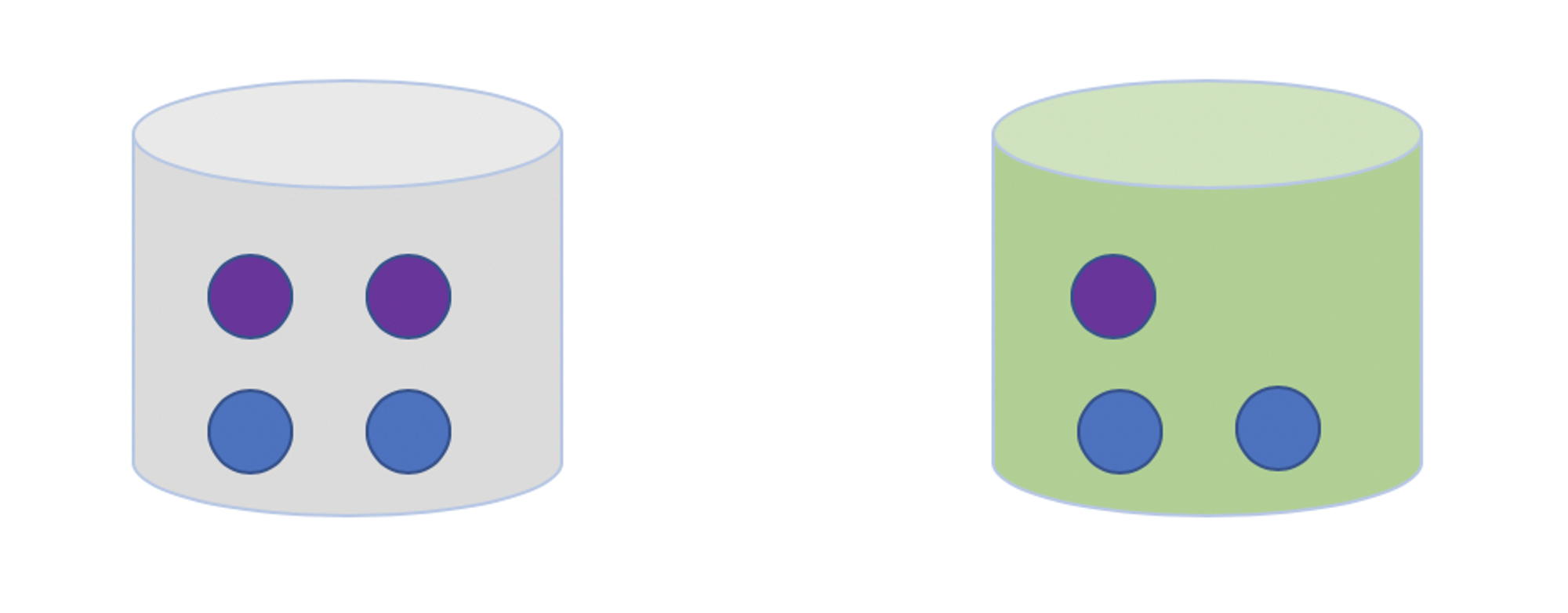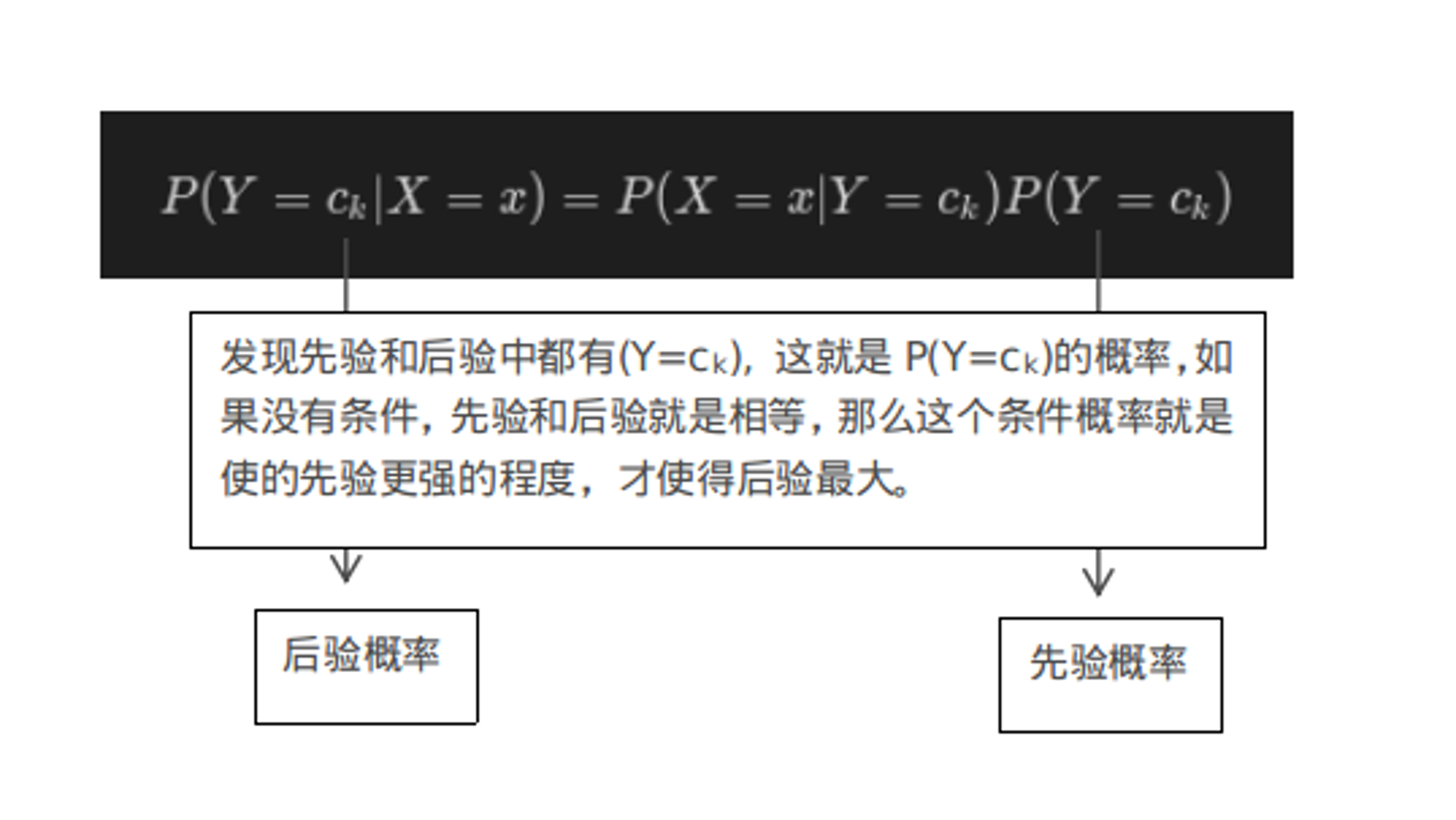V1

2023/03/23阅读：72主题：默认主题

# 机器学习算法（二）: 基于鸢尾花数据集的朴素贝叶斯(Naive Bayes)预测分类

## 1. 实验室介绍

### 1.1 实验环境

1. python3.72. numpy >= '1.16.4'3. sklearn >= '0.23.1'

### 1.2 朴素贝叶斯的介绍1. 先选择桶
2. 再从选择的桶中选择一个球

p(A,B)：表示事件A和事件B同时发生的概率。

p(B)：表示事件B发生的概率，叫做先验概率；p(A)：表示事件A发生的概率。

p(A|B)：表示当事件B发生的条件下，事件A发生的概率叫做后验概率。

p(B|A)：表示当事件A发生的条件下，事件B发生的概率。

## 2. 实验室手册

### 2.1 学习目标

1. 掌握贝叶斯公式
2. 结合两个实例了解贝朴素叶斯的参数估计
3. 掌握贝叶斯估计

### 2.2 代码流程

• Part 1. 莺尾花数据集--贝叶斯分类

• Step1: 库函数导入
• Step2: 数据导入&分析
• Step3: 模型训练
• Step4: 模型预测
• Step5: 原理简析
• Part 2. 模拟离散数据集--贝叶斯分类

• Step1: 库函数导入
• Step2: 数据导入&分析
• Step3: 模型训练&可视化
• Step4: 原理简析

## 2.3 算法实战

### 莺尾花数据集--贝叶斯分类

Step1: 库函数导入

import warningswarnings.filterwarnings('ignore')import numpy as np# 加载莺尾花数据集from sklearn import datasets# 导入高斯朴素贝叶斯分类器from sklearn.naive_bayes import GaussianNBfrom sklearn.model_selection import train_test_split

Step2: 数据导入&分析

X, y = datasets.load_iris(return_X_y=True)X_train, X_test, y_train, y_test = train_test_split(X, y, test_size=0.2, random_state=0)

Step3: 模型训练

# 使用高斯朴素贝叶斯进行计算clf = GaussianNB(var_smoothing=1e-8)clf.fit(X_train, y_train)

Step4: 模型预测

# 评估y_pred = clf.predict(X_test)acc = np.sum(y_test == y_pred) / X_test.shapeprint("Test Acc : %.3f" % acc)# 预测y_proba = clf.predict_proba(X_test[:1])print(clf.predict(X_test[:1]))print("预计的概率值:", y_proba)

Test Acc : 0.967  预计的概率值: [[1.63542393e-232 2.18880483e-006 9.99997811e-001]]

Step5: 原理简析 高斯朴素贝叶斯假设每个特征都服从高斯分布，我们把一个随机变量X服从数学期望为μ，方差为σ^2的数据分布称为高斯分布。对于每个特征我们一般使用平均值来估计μ和使用所有特征的方差估计σ^2。

### 模拟离散数据集--贝叶斯分类

Step1: 库函数导入 + Step2: 数据导入&分析 + Step3: 模型训练&可视化 + Step4: 原理简析

import randomimport numpy as np# 使用基于类目特征的朴素贝叶斯from sklearn.naive_bayes import CategoricalNBfrom sklearn.model_selection import train_test_split

Step2: 数据导入&分析

# 模拟数据rng = np.random.RandomState(1)# 随机生成600个100维的数据，每一维的特征都是[0, 4]之前的整数X = rng.randint(5, size=(600, 100))y = np.array([1, 2, 3, 4, 5, 6] * 100)data = np.c_[X, y]# X和y进行整体打散random.shuffle(data)X = data[:,:-1]y = data[:, -1]X_train, X_test, y_train, y_test = train_test_split(X, y, test_size=0.2, random_state=0)

Step3: 模型训练&预测

clf = CategoricalNB(alpha=1)clf.fit(X_train, y_train)acc = clf.score(X_test, y_test)print("Test Acc : %.3f" % acc)

Test Acc : 0.683

# 随机数据测试，分析预测结果，贝叶斯会选择概率最大的预测结果# 比如这里的预测结果是6，6对应的概率最大，由于我们是随机数据# 读者运行的时候，可能会出现不一样的结果。x = rng.randint(5, size=(1, 100))print(clf.predict_proba(x))print(clf.predict(x))
[[3.48859652e-04 4.34747491e-04 2.23077189e-03 9.90226387e-01  5.98248900e-03 7.76745425e-04]]

## 2.4 原理简析

alpha=1这个参数表示什么？

：表示第j个特征的个数。

：表示第i个样本的第j维元素。

：第i个样本的label。

### 2.4.2 朴素贝叶斯算法index 天气的好坏 是否周末 是否出门
1 出门
2 出门
3 不出门
4 出门
5 不好 出门
6 不好 不出门

a. 当出门的条件下，X_1是天气不好的概率:

b. 出门的概率

c. X_1天气不好的概率、

d. 在X_1天气不好的情况下，出门的概率:

f. 在X_1天气不好的情况下，不出门的概率:

### 2.4.3 朴素贝叶斯的优缺点V1